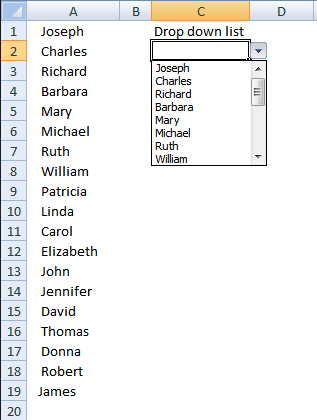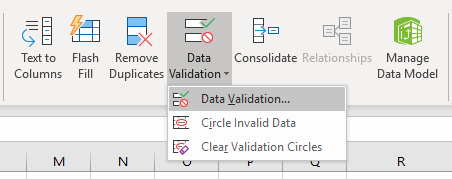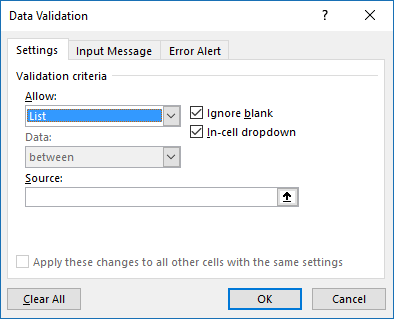Author: Oscar Cronquist Article last updated on April 25, 2019In this tutorial I am going to show you how to add values to drop down list in cell C2. This is a regular drop-down list (not form control or active-x) easily created by clicking on the "Data Validation" button on tab "Data" and then on "Data Validation...".A dialog box appears, select "List" and then click "OK" button. You don't need to specify a source range, the macro takes care of that.When a value is added, changed or deleted in column A, the drop-down list is instantly refreshed based on event code and a macro.

Explaining the vba code below

1. Find the row number of the last cell value in column A.
2. Concatenate all values in cell range into a string.
3. Add string to drop down list

VBA code

Where to copy the code?

1. Copy code below
2. Press Alt+F11
3. Insert a module
4. Paste code into code window
```Sub AddData()
Dim Lrow As Single
Dim AStr As String
Dim Value As Variant

Lrow = Worksheets("Sheet1").Range("A" & Rows.Count).End(xlUp).Row

For Each Value In Range("A1:A" & Lrow)
AStr = AStr & "," & Value
Next Value

AStr = Right(AStr, Len(AStr) - 1)

With Worksheets("Sheet1").Range("C2").Validation
.Delete
xlBetween, Formula1:=AStr
.IgnoreBlank = True
.InCellDropdown = True
.InputTitle = ""
.ErrorTitle = ""
.InputMessage = ""
.ErrorMessage = ""
.ShowInput = True
.ShowError = True
End With

End Sub
```

Explaining the vba code below

This code runs subroutine AddData whenever a cell in column A is changed.

Where to copy the code?

1. Copy code below
2. Press Alt+F11
3. Double click sheet1 in project explorer
4. Paste code into code window
```Private Sub Worksheet_Change(ByVal Target As Range)